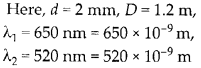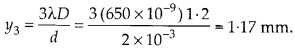Enlightened

# Question 6: NCERT Solutions for 12th Class Physics: Chapter 10-Wave Optics

• 0

Question 6: NCERT Solutions for 12th Class Physics: Chapter 10-Wave Optics

A beam of light consisting of two wavelengths, 650 nm and 520 nm is used to obtain interference fringes in a Young’s double-slit experiment.
(a) Find the distance of the third bright fringe on the screen from the central maximum for wavelength 650 nm.
(b) What is the least distance from the central maximum where the bright fringes due to both the wavelengths coincide?

Share

1. Solution:(a) Distance of third bright fringe from the central maximum for the wavelength 650 nm.(b) Let at linear distance ‘y’ from center of screen the bright fringes due to both wavelength coincides. Let n1 number of bright fringe with wavelength λ1 coincides with n2 number of bright fringe with wavelength λ2. We can writeAlso at first position of coincide, the nth bright fringe of one will coincide with (n + l) th bright fringe of other.So, the fourth bright fringe of wavelength 520 nm coincides with 5th bright fringe of wavelength 650 nm.

Check the complete chapter with solutions.

NCERT Solutions for 12th Class Physics: Chapter 10-Wave Optics

• 0